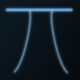Categories

# Sudoku – Take 1

While the Bulls and Cows experiment is still incomplete, this weekend I found myself trying out another interesting thing. This time it was Sudoku.

I started off writing a simple problem solver, which, given a problem gives the solution. But my intentions were different. I wanted to write a problem generator that is able to generate problems.

This is not easy as it sounds. The problem solver that I wrote was using the simple backtracking algorithm (I just want to get things working – computers today are good enough to solve these problems in negligible time).

The problem generator is more interesting because of the following constraints:

* The problem generated should have a unique solution.
* The set of givens (i.e. the digits that are present in the problem) should be a minimal set – this means that there should not be a smaller set of givens that can also generate the same unique solution.

The algorithm that I used is this:
* Fill up the first row of the matrix using random numbers.
* Generate a complete solution using this row.
* Now start off a loop analyzing which of these numbers are required and which ones are not. A number is considered as required if removing that number will result in the solution seizing from being a unique solution.

I don't know if this algorithm is right, but I expect it to guarantee that the problem generated satisfies the constraints given above.

The experiment went well and this is what I found:
“The number of givens is between 22 and 26 with a matrix of size 9×9”. Of course, as mentioned in the Wikipedia article on Sudoku, the number of givens has little or no bearing on the difficulty level. That is something that I need to analyze.

Here's the source code in C(Requires Linux/Unix because of use of system function).